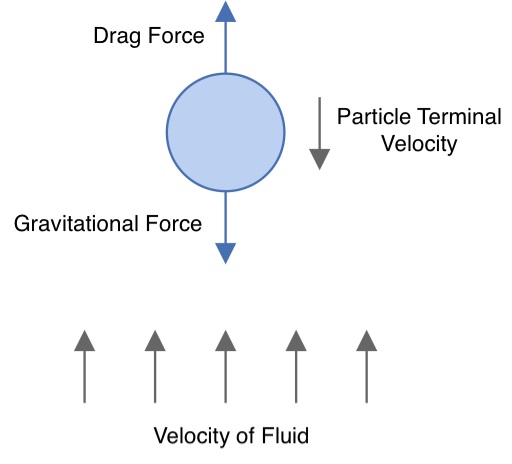# Summary

There are many operations in which two phases must be separated. These separations may be gas-liquid, gas-solid, liquid-liquid or liquid-solid, with several factors such as relative densities, gravity, fluid velocities and the shape of particles and/or droplets influence the phase separability. In this article we present the fundamentals of these separations and provide the Stoke’s, Intermediate and Newton’s formulae for calculating the terminal velocities of settling particles to analyse separation systems.

# Definitions

 : Diameter of particle or droplet : Gravitational acceleration : Reynolds Number : Velocity of the fluid relative to the droplet/particle : Terminal velocity of the droplet or particle : Viscosity of the suspending fluid : Density of the fluid : Density of the particle

# Introduction

There are many industrial processes where phase separation by gravity is required. Common examples may be removal of solids or hydrocarbons from a waste water stream, liquid knock-out from gas extraction or separation of phases after contacting during a chemical reaction. It is important to note that in the present context the term ‘particle’ is used to refer to both a solid particle and a liquid droplet.

Analysis of gravity separation is undertaken by first starting with a force balance on a single particle. The two primary forces in the system are gravity and drag. Gravity acts to pull the heavier component down while drag is experienced by a particle as it moves through a fluid, and acts in the opposite direction to the particles direction of travel. The point at which drag force and the gravity forces are equal determine the terminal velocity of the particle.The direction of movement of the particle will depend whether the density of the droplet is higher or lower than that of the bulk fluid in which it is contained. If the particle density is lower it will tend to rise, while if it is higher it will fall.

For systems in which the motion of the bulk fluid flow is in opposition to the settling direction (i.e. a vertical knockout drum), a settling particle must overcome the additional drag forces exerted by the bulk fluid motion. In these systems the settling or rising velocity of the particle must be greater than the bulk fluid velocity for separation to be possible. The closer the settling velocity is to the velocity of the bulk fluid the greater the likelihood of a particle being carried with the fluid, which is common in column type separation and is often referred to as carry-over or entrainment. To ensure that carry over is minimised the following equality must be satisfied.

Gravity separation system are often complex, and their analysis requires detailed information and computational power. However, with the aid of several simplifying assumptions a reasonable approximation of the separation characteristics of these systems can be achieved.

The primary simplifying assumption is that particles are representable as rigid spheres. This is typically an acceptable assumption for liquid-liquid and liquid-gas systems, but may not valid for systems containing solids, where particle shapes may significantly deviate from spheres (e.g. flakes, needles etc) and therefore separation behaviour may not be adequately approximated.

The secondary simplifying assumption is that there is small amounts of the particle relative to the bulk fluid in which it is suspended. As the ratio of suspended particles to bulk fluid increases there will be complex interactions between the particles, resulting in separation mechanics deviating from the simple force balance used in this model.

Equations have been derived for each fluid-flow regime (laminar, turbulent or intermediate) with the flow regime being identified through the calculation of the Reynolds number as detailed in the following section.

# Reynolds Number for a Particle in Fluid

The equation for calculating the Reynolds Number of a particle in a fluid system may be calculated as shown below.

The values of Reynolds number at which the fluid flow regimes transitional from laminar to turbulent are different than those used for traditional fluid flowing in a pipe systems. These values are listed below.

RegimeReynolds Number
Laminar< 2
Transitional2-500
Turbulent> 500

# Terminal Velocity

The key variable in gravity separation calculations is the terminal velocity of the settling particle. The terminal velocity indicates whether a heavy particle will separate against an upward fluid flow or whether a system has sufficient residence time for a particle to settle.

The calculation of terminal velocity for the three flow regimes are shown in the sections below:

## Turbulent Flow - Newton’s Law

In the turbulent flow regime Newton’s law is applied to determine the terminal velocity of a settling particle.

for 500 < Re

## Intermediate/Transitonal Flow - Intermediate Law

For the transitional flow regime the Intermediate law may be applied for the calculation of a particles terminal velocity.

for 2 < Re < 500

## Laminar Flow - Stoke’s Law

For the Laminar flow regime, Stoke’s law may be applied to calculate the terminal velocity of a particle. Gravity separation of small particles typically occurs in the laminar flow regime.

for Re < 2

## Very Fine Particles

Below a certain size, particle separation via gravity is no longer practical. As the particle size decreases, Brownian motion will become dominant relative to gravity leading to effectively, infinite settling time (certainly too long for most practical applications). In these cases additional separation equipment must be utilised such as demister pads, cyclones and membranes.

Article Tags

# Subscribe to our mailing list

 Email Name